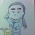## 2016年6月22日 星期三

### 訓練數學感 103 ─ 數字填空

(    ) + (    ) = (    )
(    ) + (    ) + (    ) = (    ) + (    )
(    ) + (    ) + (    ) + (    ) = (    ) + (    ) + (    )

#### 17 則留言:

1.按順序填入 1 ~ 15

1.2.兩位厲害，我還算老半天才得到等式，
6+9=15
2+7+10=5+14
3+4+8+11=1+12+13
只是沒想到有更好的答案。
請問兩位是如何解這題？

另外加分題，還有其它解嗎？

3.我承認我是先嘗試帶入1+2=3發現成立，然後再試著帶入4+5+6=7+8發現也成立。第三式再試一次發現也成立。
純粹是運氣好，哈。
我再想想有沒有其他解或一般解。

2.這讓我想到，加分題二，這樣連續填寫可以無限擴張嗎？

1.https://goo.gl/photos/SCKPu8W31TwH1bMz6
是成立的，證明如上，用打的太麻煩，直接貼照片，哈。

2.yes。沒錯，昨日就已經做過類似的證明。

3.看到了，帳號申請好，通知我一下，查了一下已經有人申請 https://oeis.org/A059270，看來要另外找。

4.行天下，你也可以預先申請一個帳號，那天說不定有機會找到個數列登記。

3.4.確實還有其他解，寫個自動賦值的試算表會快很多

1.試算表自動賦值？指巨集程式嗎？這種高階應用，太久沒碰已經忘光光。老師放暑假了嗎？

2.是巨集。我不會寫全自動的，寫了個半自動的，找到之後需要手動複製保存結果，然後再開始找下一個。
今天試運行了一下，十分鐘找到了7個，前六個第一行算式不同，第七個和第一個相同，但後面兩行完全不同。
粘貼如下：
10 1 = 11
6 5 3 = 12 2
4 14 9 8 = 13 7 15

4 11 = 15
10 12 5 = 14 13
1 3 8 6 = 7 2 9

5 1 = 6
7 13 3 = 9 14
8 2 10 11 = 4 12 15

7 6 = 13
1 10 8 = 4 15
5 2 12 9 = 14 3 11

10 4 = 14
15 1 9 = 12 13
3 7 5 6 = 11 2 8

10 3 = 13
4 11 1 = 9 7
12 5 8 6 = 2 14 15

6 7 = 13
11 1 5 = 14 13
10 2 15 3 = 4 9 8

3.七月十七日上完班就放假了。

5.嗯，這等式填空還有個特色，等號左邊全加會等於右邊全加 = 60。第七組出現兩個 13 有問題
香港的學校都這麼晚放假嗎？還是只有老師晚點放？

6.都是七月中旬才放，各校相差不大。

1.看來是港台學制差異的關係,台灣都是七八兩月暑假。